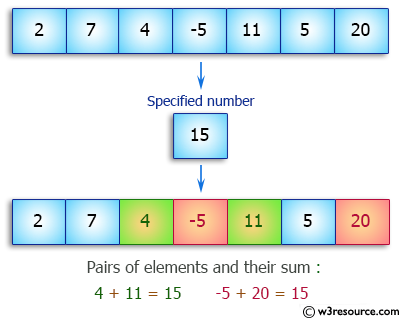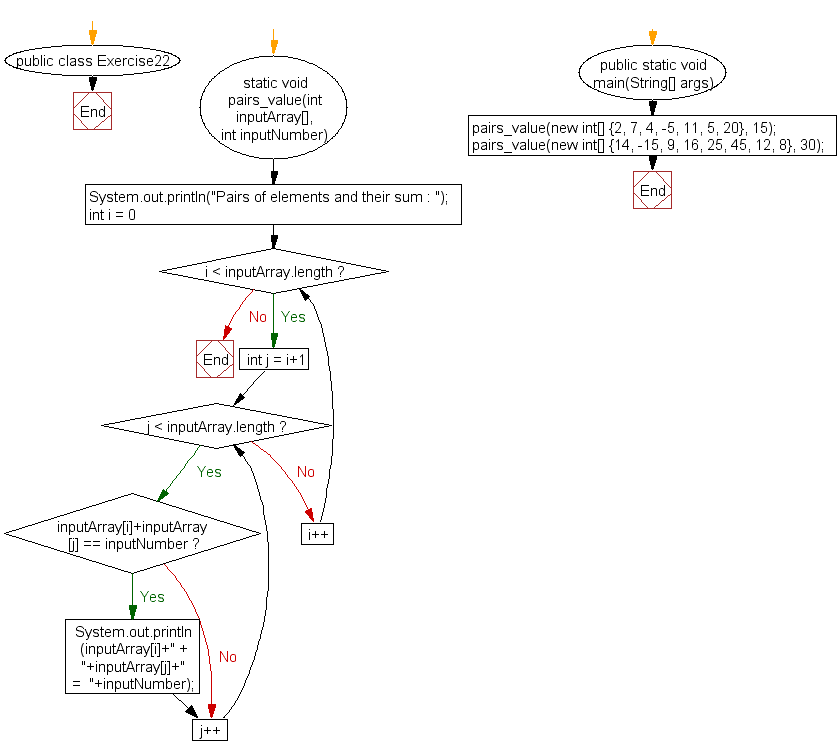﻿ Java: Find all pairs whose sum matches the value in an array# Java Array Exercises: Find all Pairs of elements in an array whose sum is equal to a specified number

## Java Array: Exercise-22 with Solution

Write a Java program to find all pairs of elements in an array whose sum is equal to a specified number.

Pictorial Presentation:Sample Solution:

Java Code :

``````public class Exercise22 {
static void  pairs_value(int inputArray[], int inputNumber)
{
System.out.println("Pairs of elements and their sum : ");

for (int i =  0; i < inputArray.length; i++)
{
for (int j  = i+1; j < inputArray.length; j++)
{
if(inputArray[i]+inputArray[j] == inputNumber)
{
System.out.println(inputArray[i]+" + "+inputArray[j]+" =  "+inputNumber);
}
}
}
}

public static void  main(String[] args)
{
pairs_value(new int[] {2, 7, 4, -5, 11, 5, 20}, 15);

pairs_value(new int[] {14, -15, 9, 16, 25, 45, 12, 8}, 30);

}
}
```
```

Sample Output:

```Pairs of elements and their sum :
4 + 11 = 15
-5 + 20 = 15
Pairs of elements and their sum :
14 + 16 = 30
-15 + 45 = 30
```

Flowchart:Visualize Java code execution (Python Tutor):

Java Code Editor:

Improve this sample solution and post your code through Disqus

What is the difficulty level of this exercise?

Test your Programming skills with w3resource's quiz.

﻿

## Java: Tips of the Day

getEnumMap

Converts to enum to Map where key is the name and value is Enum itself.

```public static <E extends Enum<E>> Map<String, E> getEnumMap(final Class<E> enumClass) {
return Arrays.stream(enumClass.getEnumConstants())
.collect(Collectors.toMap(Enum::name, Function.identity()));
}
```

Ref: https://bit.ly/3xXcFZt